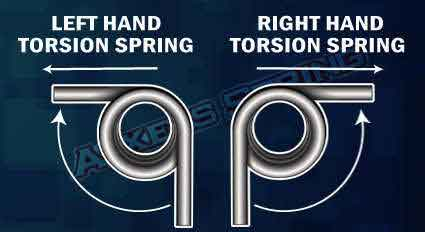<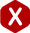# Torsion Spring Rate

Calculating the torsion spring constant can be the most critical part in torsion spring design. The definition of torsion spring rate is the amount of force per 360 degrees of radial travel. Torsion force is typically noted in pounds or newtons based on English or metric measurements. This can be further broken down into the amount of force per degree by simply dividing by 360 degrees. The torsion spring rate per degree will be useful in determining the amount of force required to torque your spring a particular amount of degrees, for example 90 degrees. Please see the example and formulas below to determine your torsion spring rate.

#### Rate Per 360 Degrees

(R) = Ed^4 / 10.8 DN

Explanation of Symbols:

• d = wire size (inches)
• D = Mean Diameter (inches)
• N = Number of active coils
• R = Rate per 360 degrees
• S = Stress (lbs / square in)
• M = Moment (inch-lbs)
• D / d = Index correction

Modulus of Elasticity "E" for common spring wires.

PSI x 10^6
• Music Wire = 30 psi
• Stainless Steel = 28 psi
• Chrome Vanadium = 30 psi
• Chrome Silicon = 30 psi
• Phosphor Bronze = 15 psi

#### Torsion Spring Rate Calculation Example:

##### Formula
Rate Per 360 degrees = Ed^4 / 10.8 DN
##### Spring Parameters
• d = 0.035 inches
• OD = 0.500 inches
• D = 0.465 inches
• N = 3 coils
• Wire Type = Music Wire
• E For Music Wire = 30 x 10^6
##### Example
Rate Per 360 Degrees = (30 x 10^6) (0.035^4) / 10.8 (0.465)(3)
Rate Per 360 Degrees = 45.01875 / 15.066
Rate Per 360 Degrees = 2.988 pounds / 360 degrees

To determine your rate per degree divide the above answer by 360

Rate Per Degree = Rate Per 360 Degrees / 360
Rate Per Degree = 2.988 / 360
Rate Per Degree = 0.0083 pounds / degree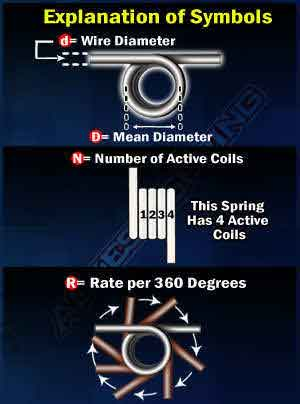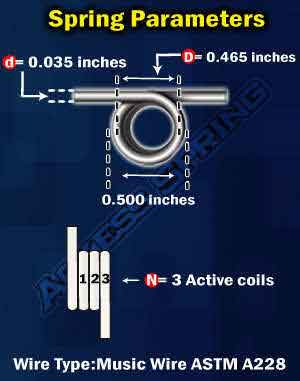Remember when designing your torsion springs, the wind direction of the torsion force is critical. You will need to know whether you have a left hand or right hand torsion spring required for your design. A left hand spring will generate torsion force in the clockwise direction, while a right hand spring will generate torsion force in a counter clock wire direction. Having the incorrect direction of wind will result in a non-functioning torsion spring. Please see the diagram to the right for an explanation.

Please note the max safe travel and the max load possible on your torsion spring. This measurement takes into account your torsion spring constant and determines how much force you can put on the spring before it shows stress, fatigue, or breaks under pressure. Please make sure when using your torsion spring rate, that you fall within the safe ranges of force to get the best results on your torsion spring design.

You can also use our online spring calculator to determine your torsion spring constant if using the torsion spring rate formula poses any issues.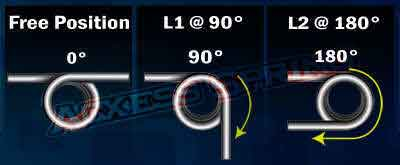#### Calculating Torsion Force (Torque)

Load = Rate Per Degree * Distanced Travel

#### Example

(L1) Load 1 = 0.0083 pounds / degree * 90 degrees